##1. Orthopole of a chord

The Wallace-Simson Lines of the extremities of any chord TT' of the circumcircle of triangle ABC pass through the orthopole S of TT' .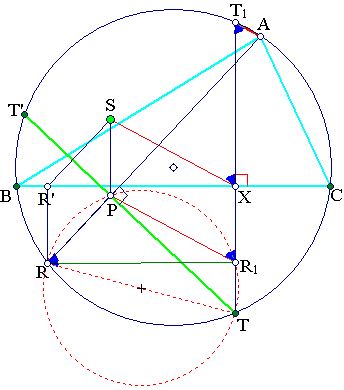Construct first the orthopole S of the chord TT'. For this draw AR orthogonal to TT', R being its second intersection point with the circumcircle. Let R' be the projection of R on side BC. The orthopoles of all lines L parallel to TT' result by translating parallel the segment RR' so that R coincides with a point of line L (see Orthopole.html ). Thus the orthopole of TT' is point S, such that PS is parallel and equal to RR', P being the intersection point of TT' with AR.
Construct now the Wallace-Simson line W(T) of T. For this project T on BC to point X and extend to define its second intersection point T1 with the circumcircle. W(T) is parallel to AT1 (see SimsonProperty.html ) and passes through X. Hence it suffices to show that XS is parallel to AT1.
This follows easily by an angle chasing argument using the cyclic quadrilateral TRPR1, resulting by projecting R onto TX.

##2. Parallel chords

Fix a point P on the circumcircle of ABC and consider all chords parallel to the tangent (t) at P. According to the previous discussion the Wallace lines W(Q1) , W(Q2) of the extremities {Q1, Q2} of these chords intersect at the orthopole OQ of the line q=Q1Q2. Since this is moved parallel to itself OQ moves on the Wallace line W(S) which is orthogonal to the direction of t (see Orthopole.html ).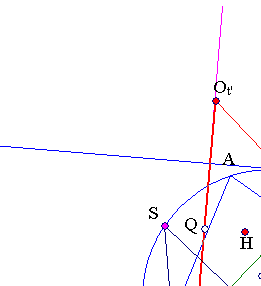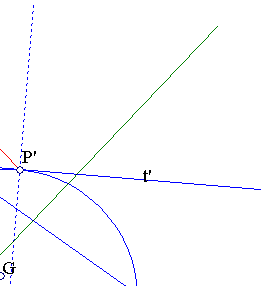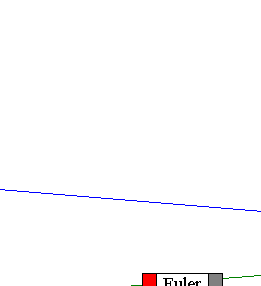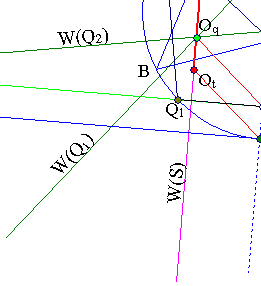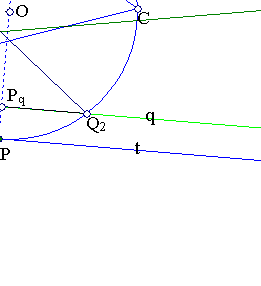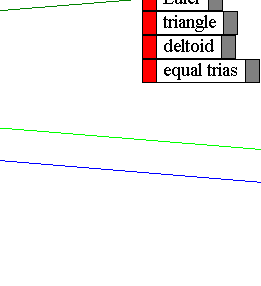Remarks
 Consider the intersection Pq of line q (moving parallel to itself) with the diameter PP'. Since the segment PqOq has constant length and direction as q moves parallel to itself, point OQ describes a segment OtOt' which is parallel and equal to the diameter PP'.
 Points Ot and Ot' are the orthopoles of the parallel lines t and t' correspondingly.
 Obviously the reverse property holds also true. If three Wallace lines {W(Q1),W(Q2),W(S)} intersect at a point Oq, then points {Q1, Q2} lie symmetrically with respect to the diameter PP' which is parallel to W(S).
 Last assertion is symmetric with respect to the three points {Q1,Q2,S} and their corresponding Wallace lines. Thus, for example, points {Q1,S} are also symmetric with respect to the diameter which is parallel to W(Q2).
 It follows that S is the intersection point of two orthogonal lines, the first from Q1 orthogonal to W(Q2) and the second from Q2 orthogonal to W(Q1).
 Hence the three Wallace lines {W(Q1),W(Q2),W(S)} are parallel to the altitudes of triangle Q1Q2S. Last triangle is an S-triangle after Lalesco. Such triangles are studied in S_Triangles.html .
 It follows that Oq is the orthopole of any one of the three side-lines of triangle Q1Q2S.
 Letting {Q1,Q2} converge to P, we see that W(P) is the Wallace line (through Ot) which is orthogonal to PS. Analogously W(P') is the Wallace line (through Ot') which is orthogonal to P'S. In particular Ot belongs to the Wallace line W(P) and Ot' to W(P').
 Because {P,P'} are diametral points the two Wallace lines {W(P),W(P')} intersect on the Euler circle (see SimsonDiametral.html ) and define on it a triangle R1R2S' antihomothetic to triangle PSP' with respect to the centroid G of triangle ABC in ratio -1/2. Lines R1S'=W(P), R2S'=W(P') and R1R2 is parallel to W(S).
 It follows further that {R1,R2} are the middles of {S'Ot, S'Ot'} and the middle Q of segment OtOt' is on the Euler circle of ABC.
 From the aforementioned homothety follows that S is symmetric to the orthocenter H of ABC with respect to the middle Q of the segment OtOt'.
 Lines W(S) envelope a deltoid studied in Deltoid.html .

##3. Chords through a fixed point

Fix a point P on the circumcircle of triangle ABC and consider all chords QP through P, Q being another point of the circumcircle. By the remarks of the previous section follows that:
 The orthopoles OQ of these chords lie all on the Wallace line W(P) of point P. OQ coincides with the intersection of W(P) with the Wallace line which is orthogonal to PQ.
 The orthopole OP of the tangent tP at P lies on W(P) and the orthopoles OQ of chords PQ take all positions in the segment Q1Q2 of W(P) determined by the orthopoles of tangents to the circumcircle at points {P1,P2}, where the diameter P1P2 is the parallel to W(P).
 The radius OP is parallel to the radius EP3 of the Euler circle. Here P3 is the intersection point of the Wallace lines W(P1) and W(P2).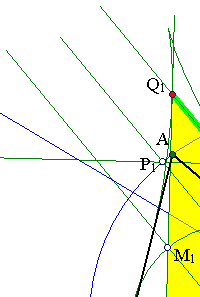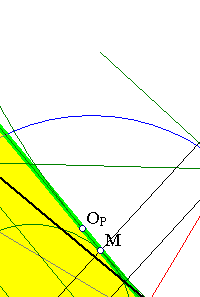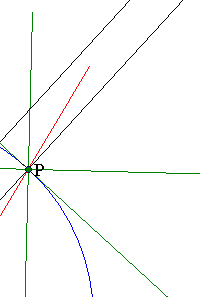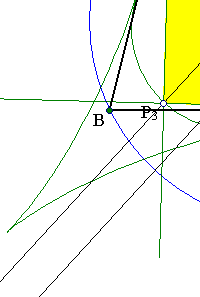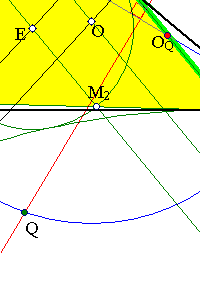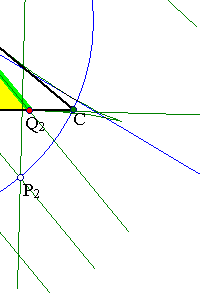Points {M1,M2,M3} are the middles of the segments {Q1P3, Q2P3, Q1Q2} on the respective Wallace lines. They are all on the Euler circle of the triangle.
These and the properties of the previous section are used in the discussion of the enveloping deltoid of the Wallace lines of the triangle ( Deltoid.html ).

##4. Deltoid generated by orthopolesConsider the Wallace lines W(P) and W(Q) of two points P, Q on the circumcircle lying closely to each other. Their intersection point O(PQ) was identified as the orthopole of line PQ. As Q tends to coincide with P, O(PQ) tends to OP the orthopole of the tangent tP at P. By the standard geometric definition of envelopes, OP is a point of the curve enveloping the Wallace lines of the triangle. This is the Deltoid displayed in the figure of the previous section. The present comment shows that this curve is generated by the various positions taken by OP as P varies on the circumcircle. This is used in the discussion in Deltoid.html .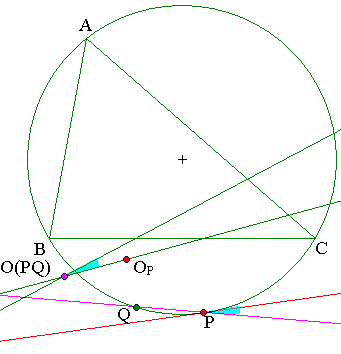Remark By the discussion in SimsonProperty2.html follows that the angle of lines (tP, QP) is equal to the angle of lines (W(P), W(Q)).

##5. Conic generated by orthopoles

It is known (see ref. , ) that taking the orthopoles OPQ of all lines PQ through a fixed point P generates a conic, which, is always an ellipse tangent to the deltoid. The conic degenerates to a line (segment) when P is on the circumcircle of triangle ABC. This conic generalizes a property discussed in Orthopole.html , according to which, for P coinciding with the circumcenter O of the triangle the conic becomes the Euler circle.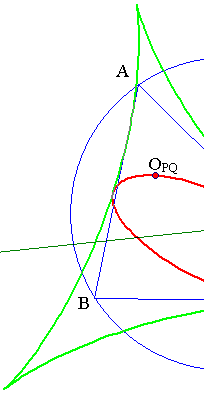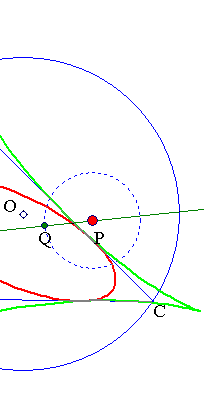Remark There is a unique position of P for which the conic generated by the orthopoles of lines through P becomes tangent to all sides of the triangle. This is discussed in De_Longchamps.html

##6. References

 Butchart, J. H. The Deltoid Regarded as the Envelope of Simson Lines The American Mathematical Monthly, Vol. 46, No. 2. (Feb., 1939), pp. 85-86.
 Gallatly William The modern geometry of the triangle London, Francis Hodgson 1913, p.49.
 Goormaghtigh, R. On Some Loci Connected with the Orthopole-Geometry The American Mathematical Monthly, Vol. 37, No. 7. (Aug. - Sep., 1930), pp. 370-371.
 Honsberger, R. Episodes in Nineteenth and Twentieth Century Euclidean Geometry. Washington DC, Math. Assoc. Ammer., 1995, pp. 106-110.
 Lalesco, T. La Geometrie du Triangle. Paris, Jacques Gabay, 1987, p. 17.
 Ramler, J. O. The Orthopole Loci of Some One-Parameter Systems of Lines Referred to a Fixed Triangle The American Mathematical Monthly, Vol. 37, No. 3, (Mar., 1930), pp. 130-136
 Van Horn, C. E. The Simson Quartic of a Triangle The American Mathematical Monthly, Vol. 45, No. 7. (Aug. - Sep., 1938), pp. 434-437.